Courses

# JEE Main Mathematics Mock - 3

## 25 Questions MCQ Test Mock Test Series for JEE Main & Advanced 2022 | JEE Main Mathematics Mock - 3

Description
This mock test of JEE Main Mathematics Mock - 3 for JEE helps you for every JEE entrance exam. This contains 25 Multiple Choice Questions for JEE JEE Main Mathematics Mock - 3 (mcq) to study with solutions a complete question bank. The solved questions answers in this JEE Main Mathematics Mock - 3 quiz give you a good mix of easy questions and tough questions. JEE students definitely take this JEE Main Mathematics Mock - 3 exercise for a better result in the exam. You can find other JEE Main Mathematics Mock - 3 extra questions, long questions & short questions for JEE on EduRev as well by searching above.
QUESTION: 1

### if the pair of lines ax2 + 2hxy + by2 + 2gx + 2fy + c = 0

Solution: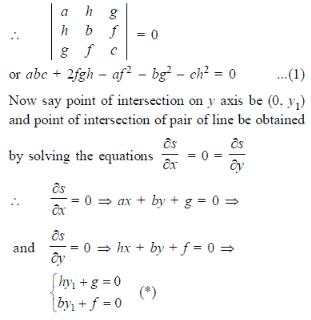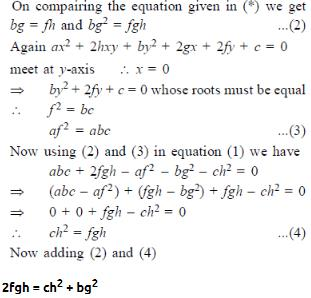QUESTION: 2

Solution:
QUESTION: 3

### The foci of the ellipse 25(x+1)2 + 9(y+2)2 = 225 are

Solution:QUESTION: 4

The product of all roots of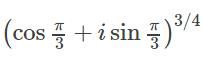is

Solution:
QUESTION: 5

The differential equation of the family of lines passing through the origin is

Solution: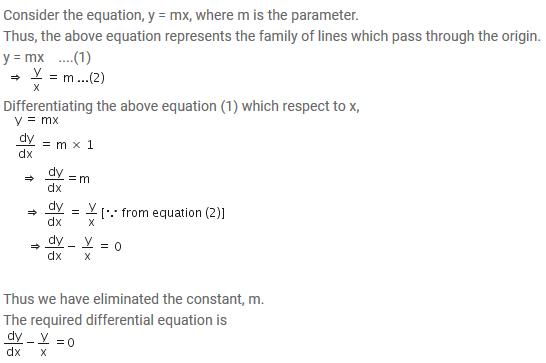QUESTION: 6
Log (x + y) - 2xy = 0, then y (0) =
Solution:
QUESTION: 7

The area bounded by the curve y = x2 - 4x, x-axis and line x = 2 is

Solution: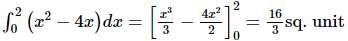QUESTION: 8

If f: R → R and g : R → R defined by f(x) = 2x + 3 and g(x) = x2 + 7, then the value of x for which f(g(x)) = 25 are

Solution:
QUESTION: 9

In the following question, a Statement of Assertion (A) is given followed by a corresponding Reason (R) just below it. Read the Statements carefully and mark the correct answer-
Assertion(A): f (x)   = log x3 and g (x)   = 3 log x are equal .
Reason(R) : Two functions f and g are said to be equal if their domains, ranges are equal and f (x)   = g (x) ∀ x in the domain .

Solution:
QUESTION: 10

Which of the following statements are true ?
(1) The amplitude of the product of complex numbers is equal to the product of their amplitudes.
(2) For any polynomial f(x) =0 with real co-efficients, imaginary roots occurs in conjugate paris.
(3) Order relation exists in complex numbers whereas it does not exist in real numbers.
(4) The value of ω used as a cube root of unity and as a fourth root of unity are different.

Solution:
QUESTION: 11

A tangent is drawn at the point (3√3 cos θ, sin θ) 0 < θ < (π/2) of an ellipse (x2/27) + (y2/1) = 1 the least value of the sum of the intercepts on the co-ordinate axes by this tangent is attained at θ =

Solution:
QUESTION: 12

In the following question, a Statement of Assertion (A) is given followed by a corresponding Reason (R) just below it. Read the Statements carefully and mark the correct answer-
Assertion (A):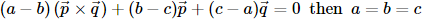Reason (R): The non zero vectors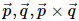are always linearly independent

Solution:
QUESTION: 13

How many numbers between 99 and 1000 can be formed from the digits 2,3,7,0,8,6 so that in each number each digit may occur once only?

Solution:
QUESTION: 14

The probabilities of solving a problem by three student A,B,C are 1/2, 1/3, 1/4 respectively. The probability that problem will be solved is

Solution:

We have, probability that A can solve the problem = P(A) = 1/2 ,
And in this way P(B) = 1/3 and P(C) = 1/4.
P(A cannot solve the problem) = 1 – P(A) = 1/2 ,
P(B cannot solve the problem) = 1 – P(B) = 1 – 1/3 = 2/3,
P(C cannot solve the problem) = 1 – P(C) = 1 – 1/4 = 3/4.
P(A, B, and C cannot solve the problem) = 1/2 x 2/3 x 3/4 = 1/4.
Therefore , P(Problem will be solve) = 1 – P(Problem is not solved by any of them)
= 1 – 1/4 = 3/4

QUESTION: 15

If two dice are thrown, find the probability of getting an odd number of on one and multiple of 3 on the other is

Solution:

Odd no. on the first die

1, 3, 5

multiple of 3 on the other die

3, 6

now let's see the combination of these two events happening simultaneously

as the question says

(1,3) , (1,6) , (3,3) , (3,6) , (5,6) , (5,3)

total no of favourable events = 6

total no of events throwing two dice simultaneously = 6² = 36

so probability = 6/36 = 1/6

QUESTION: 16

If the roots of ax2 + bx + c = 0 are α,β and roots of Ax2 + Bx + C = 0 are α + K, β + K, then B2 - 4AC/b2 - 4ac is equal to

Solution:
QUESTION: 17

Let f(x) be a polynominal function of second degree,If f(1) = f(-1) and a,b,c are in A.P., then f'(a),f'(b) and f'(c) are in

Solution:
QUESTION: 18

The total expenditure incurred by an industry under different heads is best presented as a

Solution:
QUESTION: 19
The orthocentre of a triangle whose vertices are [(2),((√3-1)/2)], ((1/2),-(1/2)) and (2,-(1/2)) is
Solution:
QUESTION: 20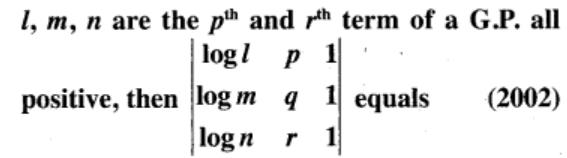Solution: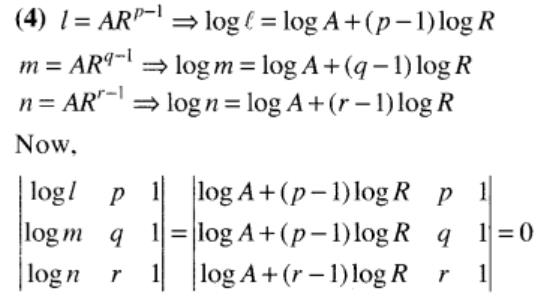*Answer can only contain numeric values
QUESTION: 21

A house of height 100 m subtends a right  angle at the window of an opposite house. If the height of the window be 64 m, then the distance between the two houses is

Solution: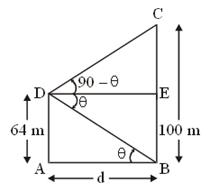*Answer can only contain numeric values
QUESTION: 22

The value of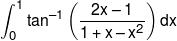is :-

Solution: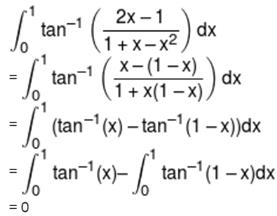*Answer can only contain numeric values
QUESTION: 23

The area (in sq. units) of the region A =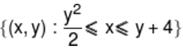is :-

Solution:
*Answer can only contain numeric values
QUESTION: 24

A line makes α/2, β/2, γ/2 angles with positive direction of coordinate axes then cosα + cosβ + cosγ equals-

Solution:
*Answer can only contain numeric values
QUESTION: 25

If a, b and c are perpendicular to b + c, c + a and a + b respectively and if |a + b| = 6, |b + c| = 8 and |c + a| = 10 then |a + b + c| =

Solution: